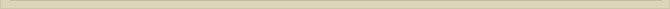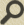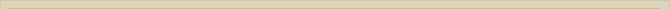## Planck’s constant

When you are interested in physics you must read “Unbelievable“!

The above analyses give the unique possibility to eliminate Planck’s constant  h as an independent natural constant.

The formula for the Planck-distance is:

We showed that the ratio between the Planck-radius and the classical radius of the electron is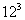.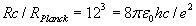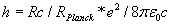The theoretical value for Planck’s (14) constant is h= 6.648982*10^-34 [Js] while the empirical measured value is h= 6.626069*10^-34 [Js].

The theoretical derived constant of Planck is a factor 1.003458 times the empirical value. The discrepancy is therefore just 0.35%. Scientists claim that this formula for Planck’s constant is merely a numerical approximation, not exact and therefore the formula is false!

Next chapter: The Deviation Between the Theoretical and Empirical Value of Planck’s constant (h)